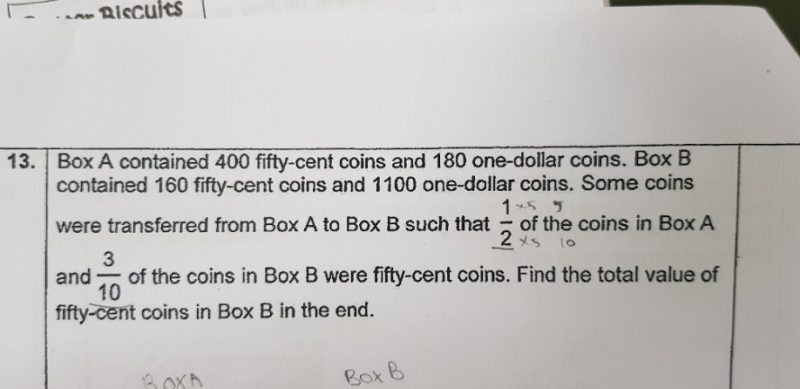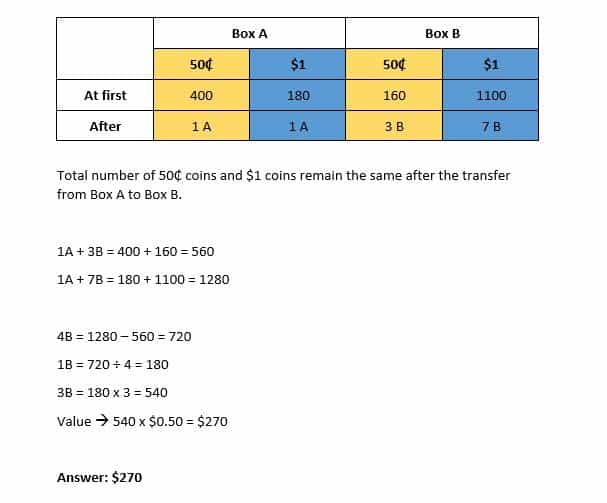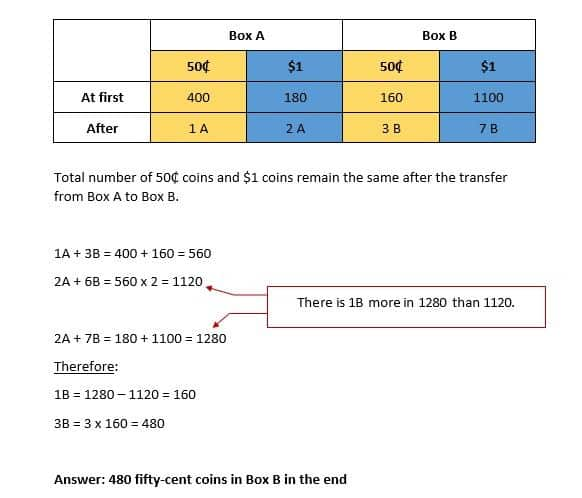QuestionHi,

DNLNMUM

Hi Priskhipo,

Correct me if i am wrong. I believe that the number of \$1 coins in the end for Box A should be 1A and not 2A. Therefore, the number of 50-cent coins in Box B is 540 and its total value is \$270.

2 Replies 0 Likes ✔Accepted AnswerDear Math Investigator,

Thank you for spotting my error.

Sorry to DNLNMum for giving you the wrong working.

I attached the amended working.

Thank you.

0 Replies 0 Likes

Hi Math Investigator,
Thanks for highlighting.
I rewrite the solution as below for reference.

After moving coins from Box A to Box B,

ratio of 50-cent to \$1 in Box A becomes 1A : 1A
ratio of 50-cent to \$1 in Box B becomes 3B : 7B

1A + 3B = 400 +160 = 560
1A + 7B = 180 +1100 = 1280
4B = 720
B = 180
3B = 180 * 3 = 540
value of 50-cent in Box B 540 * 0.50 = \$270

Thanks again Priskhipo and  Math Investigator.

Regards,

DNLNMUM

0 Replies 0 Likes

In the end —
Box A —
fifty-cents coins : 1u
one-dollar coins : 1u

Box B —
fifty-cents coins : 3p
one-dollar coins : 7p

400 + 160 = 560 (50-cents coins)
180 + 1100 = 1280 (\$1 coins)

1u + 3p ——-> 560
1u + 7p ——-> 1280

4p ——-> 1280 – 560 = 720
1p ——-> 180
1u ——-> 560 – 3 x 180 = 20
(400 – 20 + 160) x \$0.50 = \$270

Ans : \$270.

0 Replies 0 Likes2 Replies 0 LikesDear Math Investigator,

Thank you for spotting my error.

Sorry to DNLNMum for giving you the wrong working.

I attached the amended working.

Thank you.

0 Replies 0 Likes

Hi Math Investigator,
Thanks for highlighting.
I rewrite the solution as below for reference.

After moving coins from Box A to Box B,

ratio of 50-cent to \$1 in Box A becomes 1A : 1A
ratio of 50-cent to \$1 in Box B becomes 3B : 7B

1A + 3B = 400 +160 = 560
1A + 7B = 180 +1100 = 1280
4B = 720
B = 180
3B = 180 * 3 = 540
value of 50-cent in Box B 540 * 0.50 = \$270

Thanks again Priskhipo and  Math Investigator.

Regards,

DNLNMUM

0 Replies 0 Likes

Hi Priskhipo,

Thank you for the effort and answer. The working is clear and based on the answer 480 fifty-cent coins the value of fifty-cent coins in Box B is \$240 whereas the answer sheet says \$270. There is no working in answer sheet. I am not sure whether the answer in answer sheet is correct or not.

Thank you so much for the working and answer.

Regards,

DNLNMUM.

1 Reply 0 Likes

Dear DNLNMum,

I am sorry to give the wrong working. Misread the question as number of 50 cent coins is half of the number of \$1 coins. Thanks to Math Investigator for pointing out my error.

The answer of \$270 is correct. Please refer to the amended attachment.

Thank you.

0 Replies 0 Likes